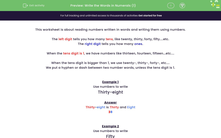# Write the Words in Numerals (1)

In this worksheet, students read numbers written in words and write them using numbers.Key stage:  KS 2

Curriculum topic:   Number: Number and Place Value

Curriculum subtopic:   Read and Write Numerals/Words to 1000

Difficulty level:#### Worksheet Overview

This worksheet is about reading numbers written in words and writing them using numbers.

The left digit tells you how many tens, like twenty, thirty, forty, fifty....etc.

The right digit tells you how many ones.

When the tens digit is 1, we have numbers like thirteen, fourteen, fifteen...etc....

When the tens digit is bigger than 1, we use twenty-, thirty-, forty-, etc....

We put a hyphen or dash between two number words, unless the tens digit is 1.

Example 1

Use numbers to write

Thirty-eight

Thirty-eight is Thirty and Eight

38

Example 2

Use numbers to write

Fifty

Fifty has no ones.

50

Example 3

Use numbers to write

Seventeen